# Validity Idea

This article gives an intuitive explanation of why smoothed conformal predictors are valid in the on-line prediction protocol: the random variables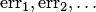are independent Bernoulli with parameter(see the article about conformal prediction for the definitions). Our heuristic explanation will ignore the difference between conformal predictors and smoothed conformal predictors.

Let us show first that the probability that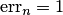is approximately. We will even show that the conditional probability, given the bag of the first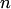observations, thatis approximately. The bag of observations determines the bag of the alphas; because of the randomness assumption all orderings of the alphas have the same probability (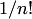). An error is made () if and only if the p-value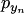corresponding to the true label is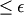if and only if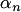is among the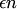largest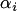. And the last event has probability.

Let us see thatare independent. It suffices to show that, for each,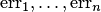are independent. Fix. It suffices to show that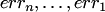are independent. We will show that they are independent given the bag of the firstobservations. We already know that the probability of, given the bag, is. Draw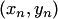from the bag. The same argument shows that the probability of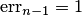, given the bag and, is. Therefore, the probability of, given the bag and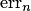, is. In the same way we obtain thatis the probability of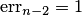, given the bag and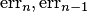.

For a formal proof see, e.g., Vovk et al. (2005), Theorem 8.1. For a less general but easier to understand proof, see Vovk (2002), Theorem 1.